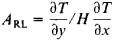Righi-Leduc Effect

Righi-Leduc effect

[′rē·gē lə′du̇k i‚fekt]
(physics)
The phenomenon wherein, if a magnetic field is applied at right angles to the direction of a temperature gradient in a conductor, a new temperature gradient is produced perpendicular to both the direction of the original temperature gradient and to the magnetic field. Also known as Leduc effect.
McGraw-Hill Dictionary of Scientific & Technical Terms, 6E, Copyright © 2003 by The McGraw-Hill Companies, Inc.
The following article is from The Great Soviet Encyclopedia (1979). It might be outdated or ideologically biased.

Righi-Leduc Effect

a thermomagnetic effect. When an electrically open conductor in which a temperature gradient and a heat flow are maintained is placed in a constant magnetic field H, there is established a difference in temperature in the direction perpendicular to the primary heat flow and the magnetic field. This phenomenon is called the Righi-Leduc effect and was discovered almost simultaneously in 1887 by the Italian physicist A. Righi and the French physicist S. Leduc. Like the Hall effect, it is due to the curvature of electrons in the magnetic field. The Righi-Leduc effect is measured by the Righi-Leduc coefficientHere, ∂T/∂x is the primary temperature gradient and ∂T/y is the temperature gradient resulting from the application of the magnetic field.

Stated most simply, ARL = eτ/m*c, where τ is the mean free time of an electron, e is the electron’s charge, m* is the effective mass, and c is the speed of light. The sign of ARL depends on the sign of the charge carriers; ARL < 0 for electrons, and ARL > 0 for holes. There exists a simple approximate relation between ARL, the Hall constant R, and the conductivity σ: ARL = σR.

The Righi-Leduc effect, like other thermomagnetic effects, has been studied more thoroughly in semiconductors than in metals. It is made use of in the investigation of the mobility of charge carriers in a solid.

M. I. KAGANOV